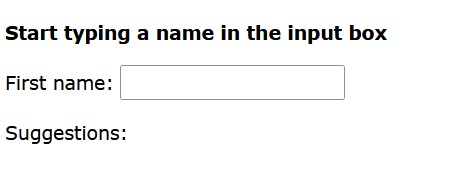# AJAX & PHP

## What exactly is AJAX?

AJAX is an acronym for Asynchronous JavaScript and XML. With the help of XML, HTML, CSS, and Java Script, AJAX is a new way to make web apps that are better, faster, and more interactive.

Conventional web applications use synchronous requests to send and receive information from and to the server. This means that when you fill out a form and hit “Submit,” the server sends you to a new page with new information.

With AJAX, when you click “Submit,” JavaScript will send a request to the server, read the response, and update the screen. In a perfect world, the user would never even know that anything was sent to the server.

AJAX Tutorial has everything you need to know about AJAX.

## An Example of AJAX & PHP

To show how easy it is to get information from a database using Ajax and PHP, we’ll build MySQL queries on the fly and show the results on “result.html.” But before we move on, let’s set the stage. Use the following command to make a table.

CREATE TABLE `student_class` (
`name` varchar(50) NOT NULL,
`age` int(11) NOT NULL,
`sex` varchar(1) NOT NULL,
`rollnum` int(11) NOT NULL,
PRIMARY KEY (`name`)
)

NOTE: We’re going to assume you have the right permissions to do the following MySQL operations.

Now dump the data into this table using this SQL statements.

INSERT INTO `student_class` VALUES (‘Ram’, 120, ‘m’, 50);
INSERT INTO `student_class` VALUES (‘Shaym’, 75, ‘m’, 44);
INSERT INTO `student_class` VALUES (‘Shiv’, 45, ‘m’, 87);
INSERT INTO `student_class` VALUES (‘Sita’, 22, ‘f’, 72);
INSERT INTO `student_class` VALUES (‘Radha’, 27, ‘f’, 20);
INSERT INTO `student_class` VALUES (‘Ganesh’, 35, ‘m’, 60);

### AJAX PHP Example

When a user types a character into the input field in the above example, a function called “showname()” is run.

The onkeyup event is what makes the function run.

#### Client Side HTML file

Here is the code for HTML:

<!DOCTYPE html>
<html>
<script>
function showname(str) {
if (str.length == 0) {
document.getElementById(“txtname”).innerHTML = “”;
return;
} else {
var xmlhttp = new XMLHttpRequest();
if (this.readyState == 4 && this.status == 200) {
document.getElementById(“txtname”).innerHTML = this.responseText;
}
}
xmlhttp.open(“GET”, “name.php?q=”+str, true);
xmlhttp.send();
}
}
</script>
<body>

<p><b>Start typing a name in the input box:</b></p>
<form action=””>
<label for=”fname”>First name:</label>
<input type=”text” id=”fname” name=”fname” onkeyup=”showname(this.value)”>
</form>
<p>Suggestions: <span id=”txtname”></span></p>

</body>
</html>

#### Code explanation:

First, make sure that the field is not empty (str.length == 0). If it is, delete the text in the textname placeholder and end the function.

If the field isn’t empty, however, do the following:

Create an XMLHttpRequest object
Make the function that will be run when the server response is ready.
Send the request to a PHP file on the server (name.php)
Notice that the q parameter is added to the URL (name.php?q=”+str).
The input field’s text is stored in the str variable.

#### Output### name.php example

#### Server Side PHP file

<?php
// Array with names
\$naam[] = “Arjun”;
\$naam[] = “Bharat”;
\$naam[] = “Chandra”;
\$naam[] = “Dev”;
\$naam[] = “Eisha”;
\$naam[] = “Faran”;
\$naam[] = “Guna”;
\$naam[] = “Hetal”;
\$naam[] = “Indra”;
\$naam[] = “Jiva”;
\$naam[] = “Kumar”;
\$naam[] = “Liger”;
\$naam[] = “Neha”;
\$naam[] = “Ophelia”;
\$naam[] = “Pinki”;
\$naam[] = “Anni”;
\$naam[] = “Raja”;
\$naam[] = “Chandu”;
\$naam[] = “Dharam”;
\$naam[] = “Shiv”;
\$naam[] = “Krishna”;
\$naam[] = “Ram”;
\$naam[] = “Hanuman”;
\$naam[] = “Laxman”;
\$naam[] = “Veer”;
\$naam[] = “Lisa”;
\$naam[] = “Elizabeth”;
\$naam[] = “Alok”;
\$naam[] = “Rohit”;
\$naam[] = “Vicky”;

// get the q parameter from URL
\$q = \$_REQUEST[“q”];

\$name = “”;

// lookup all hints from array if \$q is different from “”
if (\$q !== “”) {
\$q = strtolower(\$q);
\$len=strlen(\$q);
foreach(\$naam as \$name) {
if (stristr(\$q, substr(\$name, 0, \$len))) {
if (\$name === “”) {
\$name = \$name;
} else {
\$name .= “, \$name”;
}
}
}
}

// Output “no suggestion” if no hint was found or output correct values
echo \$name === “” ? “no suggestion” : \$name;
?>

People also search
Scroll to Top